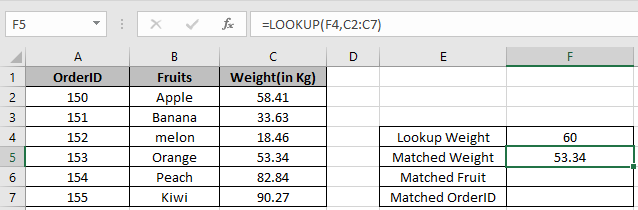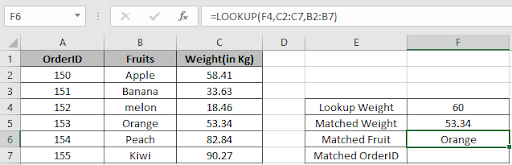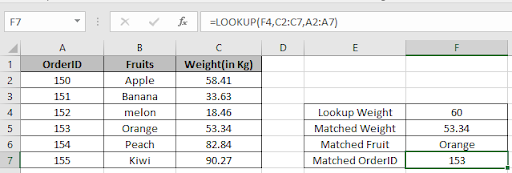# How to use the LOOKUP function in Excel

LOOKUP function finds the estimated value in the range and returns the value from the range or corresponding value.
Syntax:

=LOOKUP (lookup_value, lookup_vector, [result_vector])

lookup_value: value to look up for
lookup_vector: array where the function looks for the lookup_value.
[result vector]:[optional] if the return array is different from the lookup array.
Let’s understand this by using it in Example
Here we have some OrderID, Fruits and their weight.Use the LOOKUP formula to find the estimated value from the Weight column.

=LOOKUP(60, C2:C7)

60: lookup value
C2:C7: lookup rangeAs you can see the nearest weight in the cell.
Now we wish to find more details from Matched weight like fruit name
Use the formula to find the fruit.

=LOOKUP(F4,C2:C7,B2:B7)As you can see the Fruit name corresponding to nearest weight in the cell.
Use the formula to find the OrderID.

=LOOKUP(F4,C2:C7,B2:B7)As you can see, the OrderID corresponding to the nearest weight in the cell.

Hope you understood how to use the LOOKUP function in Excel. Explore more articles on lookup value here. Please feel free to state your queries below in the comment box.

Related Articles:

How to Retrieve Latest Price in Excel

Popular Articles:

How to use the VLOOKUP Function in Excel

How to use the COUNTIF function in Excel 2016

How to Use SUMIF Function in Excel

Terms and Conditions of use

The applications/code on this site are distributed as is and without warranties or liability. In no event shall the owner of the copyrights, or the authors of the applications/code be liable for any loss of profit, any problems or any damage resulting from the use or evaluation of the applications/code.